# Civil Engineering - Highway Engineering

### Exercise :: Highway Engineering - Section 3

6.

The correct formula for calculating superelevation for the hill roads, is

 A.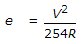B.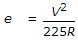C.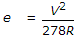D.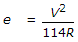Explanation:

No answer description available for this question. Let us discuss.

7.

The inventor of road making as a building science, was

 A. Sully B. Tresguet C. Telford D. Macadam.

Explanation:

No answer description available for this question. Let us discuss.

8.

An upgrade g1% is followed by a downgrade g2%. The equation of the parabolic curve of length L to be introduced, is given by

 A.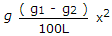B.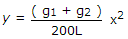C.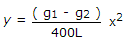D.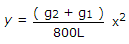Explanation:

No answer description available for this question. Let us discuss.

9.

Area of steel required per metre width of pavement for a length of 20 m for design wheel load 6300 kg and permissible stress in steel 1400 kg/cm2, is

 A. 70 kg/sq cm B. 80 kg/sq cm C. 90 kg/sq cm D. 100 kg/sq cm

Explanation:

No answer description available for this question. Let us discuss.

10.

Design of horizontal curves on highways, is based on

 A. design speed of vehicles B. permissible friction on the road surface C. permissible centrifugal ratio D. permissible maximum super-elevation E. all the above.